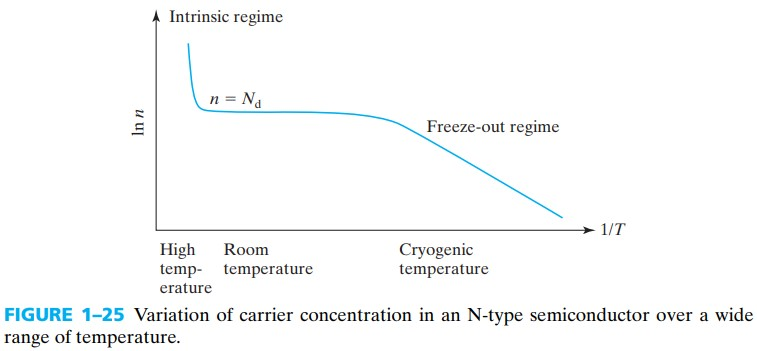# Electrons and Holes in Semiconductors

\begin{align*} \newcommand{\dif}{\mathop{}\!\mathrm{d}}\\ \newcommand{\p}{\partial}\\ \newcommand{\bd}{\boldsymbol}\\ \end{align*}

# Vacabulary

1. crystalline
2. X-ray diffraction

# 1.1 Silicon Srystal Structure

• A crystalline solid consists of atoms arranged in a repetitive structure, which can be determined by means of X-ray diffraction and electron microscopy.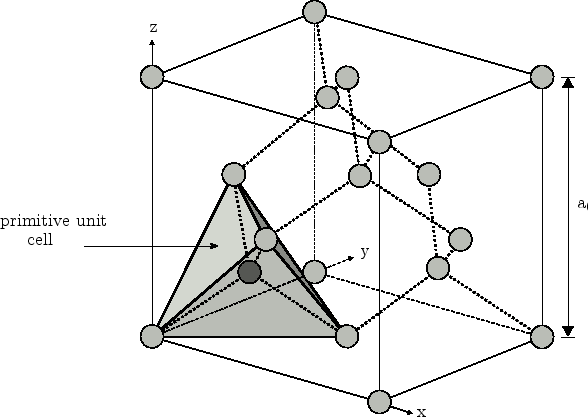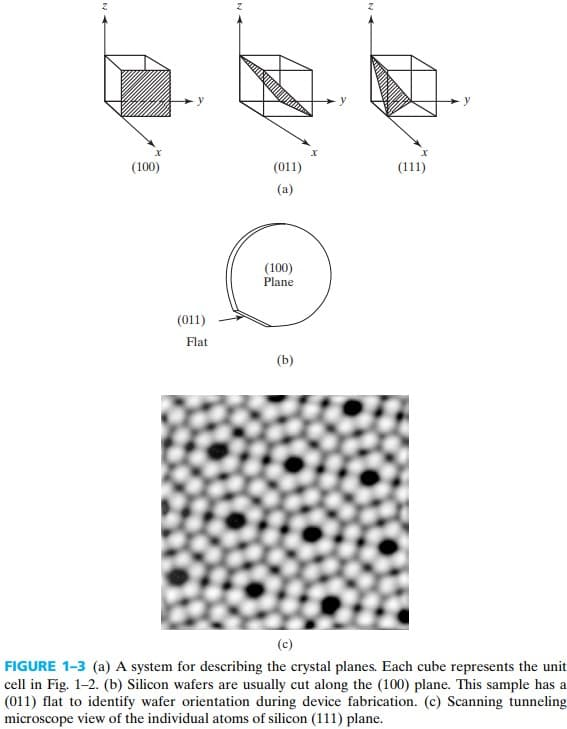# 1.2 Bond Model of Electrons and Holes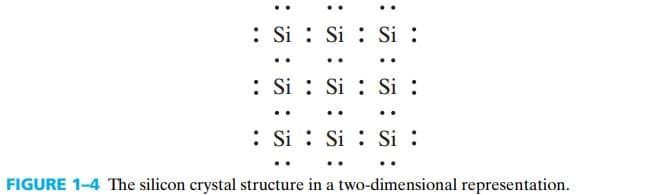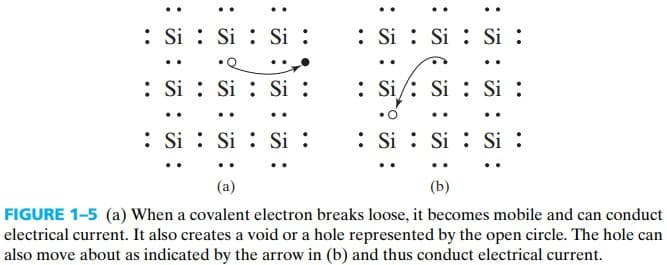• donors：group Ⅴ elements, donate electrons and generate N-type semiconductors, e.g. As
• acceptors：group Ⅲ elements, accept electrons and generate P-type semiconductors, e.g. B

$E_\text{ion} = \frac{m_0 q^4}{8 \varepsilon_0^2 h^2} = 13.6 eV$

As for silicon, we make some modification:

1. replace the permittivity of free space $\varepsilon_0$ with $\varepsilon_r \varepsilon_0=12 \varepsilon_0$ (12 is the relative permittivity of silicon)
2. replace the free electron mass $m_0$ with a electron effective mass $m_n$

After the modification, 杂质在硅中的电离能 the ionization energy is about 50 meV, 大大小于热能，所以我们可以认为室温下杂质原子完全电离。

# 1.3 Energy Band Model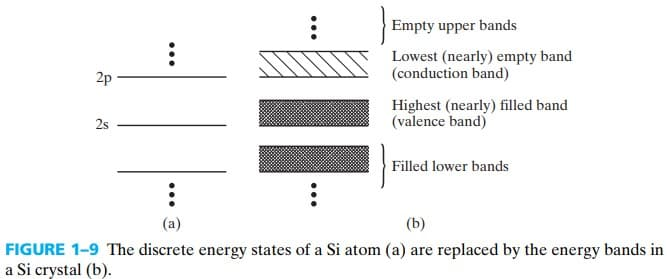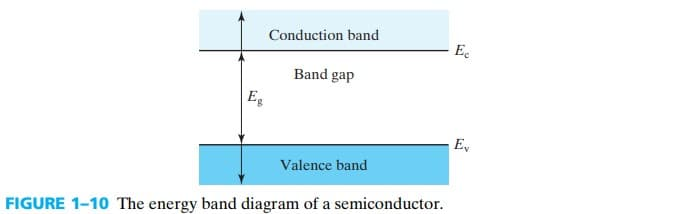band-gap energy 可以通过 measuring the absorption of light 来间接测量，当 $hv=E_g$ 时，光吸收比较强（具体可看 禁带宽度的测量 - 百度文库 (baidu.com) ），比如说：

EXAMPLE 1-1: Measuring the Band-Gap Energy
If a semiconductor is transparent to light with a wavelength longer than 0.87 µm, what is its band-gap energy?

SOLUTION:
\begin{aligned} h v &= h \frac{c}{\lambda} \\ &= 6.63 \times 10^{-34} {\rm (J\cdot s)}\cdot \frac{3\times 10^8 {\rm m/s}}{0.87 \unicode{x03BC} {\rm m }}\\ &=1.42 {\rm eV} \end{aligned}
Therefore, the band gap is 1.42 eV.

USEFUL RELATIONSHIP:
$$hv ({\rm eV})=\frac{1.24}{\lambda (\unicode{x03BC} {\rm m })}$$

Semiconductor InSb Ge Si GaAs GaP ZnSe Diamond
$E_g$(eV) 0.18 0.67 1.12 1.42 2.25 2.7 6.0

## 1.3.1 Donors and Acceptiors in the Band Model

donors 的电离能比较小，也就是说 donor level $E_d$ 和 Conduction band 离得比较近；同理，acceptor level $E_a$ 和 Valence band 离得比较近。得到的能带图如下 fig 1-12Donors Acceptors
Dopant Sb P As B Al In
Ionization energy(mV) 39 44 54 45 57 160

# 1.4 Semiconductors, Insulators, and Conductors

The differences among semiconductors, insulators, and conductors are:

• Semiconductor has a nearly filled valence band and a nearly empty conduction band separated by a band gap.
• Insulator is similar to semiconductor except for a larger $E_g$
• Conductor has a partially filled conduction band.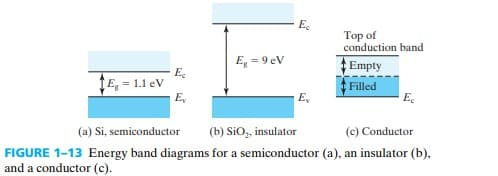# 1.5 Electrons and Holes

## 1.5.1 Effective Mass

\begin{align} a&=\frac{-q \mathscr{E}}{m_n} \qquad \text{electrons}\\ a&=\frac{q \mathscr{E}}{m_p} \qquad \text{holes} \end{align}

Si Ge GaAs InAs AlAs
$m_n/m_0$ 0.26 0.12 0.068 0.023 2.0
$m_p/m_0$ 0.39 0.30 0.50 0.30 0.3

$\text{Effective mass } m^* \equiv \frac{\hbar^2}{\dif^2 E/ \dif \bd{k}^2}$

# 1.6 Density of States

$D(E) \equiv \frac{\text{number of states in } \Delta E}{\Delta E \times \text{volume}} \;\mathrm{cm^{-3}\cdot eV^{-1}}$

$D_c(E) = \frac{8 \pi m_n \sqrt{2 m_n(E-E_c)}}{h^3}, \quad E \geq E_c\\ D_v(E) = \frac{8 \pi m_p \sqrt{2 m_p(E_v-E)}}{h^3}, \quad E \leq E_v$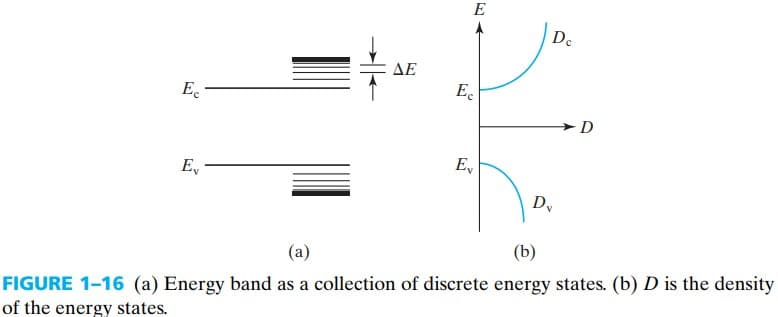# 1.7 Thermal Equilibrium and the Fermi Function

thermal equilibirium 并不是说电子就静止在导带上，它依然有一定概率再跃迁到其他能级，因此这是一种动态平衡。

$f(E)=\dfrac{1}{1+e^{(E-E_F)/kT}}\\$

$E$ 是某一能级，$E_F$ 是 Fermi energy 或 Fermi level. 在一定温度下费米能级是固定的。在费米能级处，$f(E)=0.5$，如果费米能级越高，显然，电子占据高能级的概率就越大（也就是电离程度越大）

$f(E) \approx - e^{-(E - E_F)/kT}$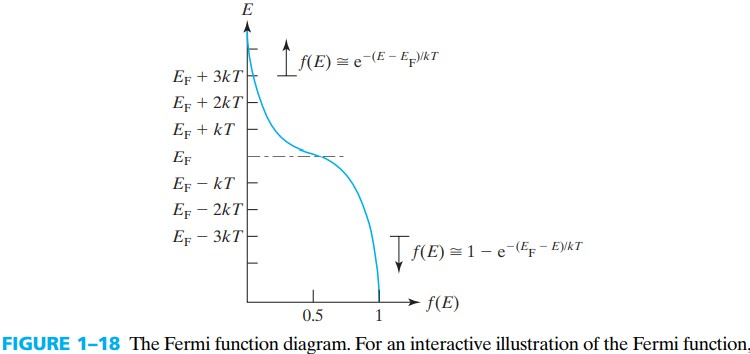$1-f(E)\approx e^{-(E_F - E)/kT}$

# 1.8 Electron and Hole Concentrations

$n = \int_{E_1}^{E_2} f(E) D(E) \dif E$

\begin{aligned} n &= \int_{E_c}^{\infty} f(E) D(E) \dif E\\ &= \frac{8 \pi m_n \sqrt{2 m_n}}{h^3} \int_{E_c}^{\infty} \sqrt{E-E_c} e^{-(E-E_F)/kT} \dif E\\ &= \frac{8 \pi m_n \sqrt{2 m_n} }{h^3} (kT)^{3/2} e^{-(E_c-E_F)/kT} \int_{0}^{\infty} \sqrt{E-E_c} e^{-(E-E_c)/kT} \dif (E-E_c) \end{aligned}

$n=N_c e^{-(E_c-E_F)/kT} \tag{1.8.5}\\ N_c \equiv 2 \left[\frac{2\pi m_n kT}{h^2}\right]^{3/2}$

$N_c$ is called the effective density of states（有效态密度）。 It is as if all the energy states in the conduction band were effectively squeezed into a single energy level, $E_c$, which can hold $N_c$ electrons (per cubic centimeter).

空穴也有类似的公式：

$p = N_v e^{-(E_F-E_v)/kT} \tag{1.8.8}\\ N_v \equiv 2 \left[\frac{2\pi m_p kT}{h^2}\right]^{3/2}$

常用的 $N_c,N_v$ 取值如下：

$T=300K$ Ge Si GaAs
$N_c {\rm (cm^{-3})}$ $1.04\times10^{19}$ $2.8\times10^{19}$ $4.7\times 10^{17}$
$N_v {\rm (cm^{-3})}$ $6.0\times 10^{18}$ $1.04\times 10^{19}$ $7.0\times 10^{18}$

By the way, 这节中的 $m_n,m_p$ 是 density-of-states effective mass，而 1.5 节中的是 conductivity effective mass，这俩个有效质量在数值上略有不同，但貌似考试中当成是一个东西。

## 1.8.1 Fermi Level and the Carrier Concentrations

注意到费米能级离 $E_c$ 或 $E_v$ 越近，导带 或 价带上的电子/空穴就越多。那么反过来，参杂浓度越大，费米能级离 $E_c$ 或 $E_v$ 越近。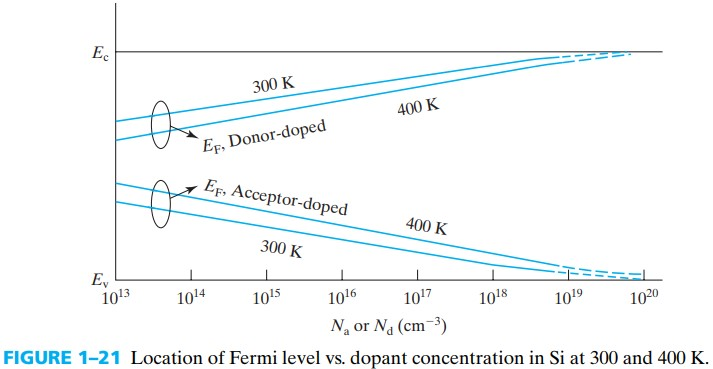EXAMPLE 1–3 Finding the Fermi Level in Si（计算费米能级）
Where is $E_F$ located in the energy band of silicon, at 300K with $n = 10^{17} \text{cm}^{–3}$? And for $p = 10^{14} \text{cm}^{–3}$?

\begin{aligned} E_c-E_F &= kT \cdot \ln(N_c/n)\\ &=0.026 \ln(2.8 \times 10^{19}/10^{17})\\ &=0.146 \text{ eV} \end{aligned} Therefore, $E_F$ is located at 146 meV below $E_c$.

\begin{aligned} E_F - E_v &= kT \cdot \ln(N_v/p)\\ &=0.026 \ln(1.04\times10^{19}/10^{14})\\ &=0.31 \text{ eV} \end{aligned} Therefore $E_F$ is located at 0.31 eV above $E_v$.

## 1.8.2 The np Product and the Intrinsic Carrier Concentration

$np=N_c N_v e^{-(E_c-E_v)/kT}=N_c N_v e^{-E_g/kT}$

$np=n_i^2 \tag{1.8.11}\\ n_i=\sqrt{N_c N_v} e^{-E_g/2kT}$

$n_i$ 称为 intrinsic carrier concentration，”intrinsic” 的中文是「本征的」，指的是无掺杂的情况。从这点可以看出，无论掺杂浓度多少，$np=n_i^2$ 只与温度有关。这个式子有点像高中的离子平衡。

300K Si GaAs Ge
$n_i$ $1.5\times 10^{10} \text{cm}^{-3}$ $1.8\times 10^{6} \text{cm}^{-3}$ $2.4\times 10^{13} \text{cm}^{-3}$

# 1.9 General Theogy of n and p

shallow donor and acceptor levels 同样满足 Fermi-Dirac 分布，所以上面的式子同样适用于施主、受主能级。

$n=N_d \text{ or } p=N_a$

\begin{aligned} &\begin{cases} n+N_a = p+N_d\\ np=n_i \end{cases}\\ \Rightarrow &\begin{cases} n=\frac{N_d-N_a}{2}+\left[ \left( \frac{N_d-N_a}{2} \right)^2+n_i^2 \right]^{1/2}\\ p=\frac{N_a-N_d}{2}+\left[ \left( \frac{N_a-N_d}{2} \right)^2+n_i^2 \right]^{1/2} \end{cases} \end{aligned} \tag{1.9.2}\label{1.9.2}
1. 若 $N_d - N_a \gg n_i$，即 N型，则可以忽略 $n_i$ 项，得到： \begin{aligned} n&=N_d-N_a\\ p&=n_i^2/n \end{aligned} \tag{1.9.3}
2. 若 $N_a - N_d \gg n_i$，即 N型，则可以忽略 $n_i$ 项，得到：

\begin{aligned} p&=N_a-N_d\\ n&=n_i^2/p \end{aligned} \tag{1.9.5} 我们将上面两种杂质相互抵消的现象称为：dopant compensation 杂质补偿.

# 1.10 Carrier Concentrations at Extremely High and Low Temperatures

$n=p=n_i$

$n=\left[\frac{N_cN_d}{2}\right]^{1/2} e^{-(E_c-E_d)/2kT}$